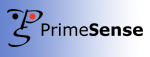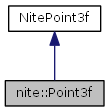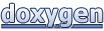# NiTE 2.0: nite::Point3f Class Reference

#### NiTE 2.0NiTE 2.0
nite::Point3f Class Reference

`#include <NiTE.h>`

Inheritance diagram for nite::Point3f:[legend]

List of all members.

## Public Member Functions

Point3f ()
Point3f (float x, float y, float z)
Point3f (const Point3f &other)
bool operator!= (const Point3f &other) const
Point3foperator= (const Point3f &other)
bool operator== (const Point3f &other) const
void set (float x, float y, float z)

## Detailed Description

Encapsulates a single point in 3D space, storing the x/y/z coordinates as floating point numbers.

Can also be used to represent a three dimensional vector.

## Constructor & Destructor Documentation

 nite::Point3f::Point3f ( ) ` [inline]`

Default Constructor, creates a point with all three coordinate values set to 0.0

 nite::Point3f::Point3f ( float x, float y, float z ) ` [inline]`

Constructor. Creates a point from three given coordinates.

Parameters:
 [in] x X coordinate of point to be created [in] y Y coordinate of point to be cretaed [in] z Z coordinate of point to be created
 nite::Point3f::Point3f ( const Point3f & other ) ` [inline]`

Copy Constructor. Creates a new point that has the same coordinates as an existing point.

Parameters:
 [in] other An existing point to be copied.

## Member Function Documentation

 bool nite::Point3f::operator!= ( const Point3f & other ) const` [inline]`

Inequality operator. Tests two points to see if they are different.

Parameters:
 [in] other One of two points to test
Returns:
True if either x, y, or z coordinate of first point differs from the matching coordinate of the second point.
 Point3f& nite::Point3f::operator= ( const Point3f & other ) ` [inline]`

Assignment operator. Sets all coordinates of the point on the left to be equal to the coordinate values of the point on the left.

Parameters:
 [in] other Point to copy coordinates from
Returns:
Reference to a point containing a copy of the input points coordinates.
 bool nite::Point3f::operator== ( const Point3f & other ) const` [inline]`

Equality operator. Tests two points to determine whether they have matching coordinate values.

Parameters:
 [in] other One of the points to be tested against
Returns:
True if all three coordinate values of left point match all three coordinate values of right point.
 void nite::Point3f::set ( float x, float y, float z ) ` [inline]`

Setter function for all coordinates of the point.

Parameters:
 [in] x Desired new X coordinate of the point. [in] y Desired new Y coordinate of the point. [in] z Desired new Z coordinate of the point.

The documentation for this class was generated from the following file:
Generated on Thu Jun 6 2013 17:48:15 for NiTE 2.0 by1.7.5.1